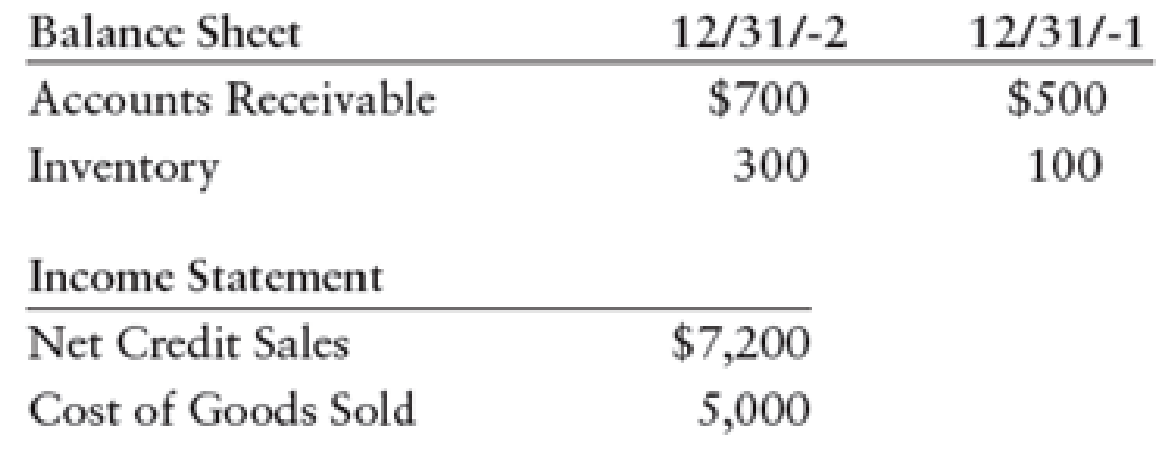Chapter 15, Problem 1CP

Chapter
Section
Textbook Problem

John Byers owns and operates Byers Building Supplies. The following information was taken from his financial statements:All sales are made on account.REQUIREDBased on the above information, on average, approximately how many days pass from the time Byers purchases inventory until he receives cash from customers?

To determine

Identify the average days to convert inventory to cash.

Explanation

Operating cycle:

The sequence of activities which includes the investment of cash to purchase goods, sell goods, generate revenues, receive cash, and repay the expenses, are referred to as operating cycle.

Calculate average days to convert inventory to cash.

Average days to convert inventory to cash) =(Average days to sell inventory + Average days to collect receivables)=14.6days + 30.4days=45days

Working notes:

(1) Calculate inventory turnover ratio.

Inventory turnover =Cost of goods soldAverage inventory=$5,000($100+$300)2=$5,000\$200=25times

(2)Calculate average days to sell inventory

Still sussing out bartleby?

Check out a sample textbook solution.

See a sample solution

The Solution to Your Study Problems

Bartleby provides explanations to thousands of textbook problems written by our experts, many with advanced degrees!

Get Started